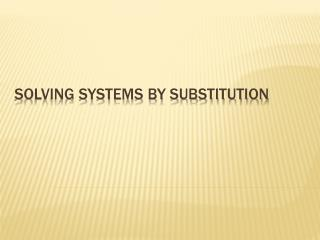DownloadDownload PresentationSolving Systems by Substitution

# Solving Systems by Substitution

Download Presentation## Solving Systems by Substitution

- - - - - - - - - - - - - - - - - - - - - - - - - - - E N D - - - - - - - - - - - - - - - - - - - - - - - - - - -
##### Presentation Transcript

1. Solving Systems by Substitution

2. ***Recall from yesterday*** Step 1 – rearrange one/both equations so they equal to ‘y’ Step 2 – set the 2 equations equal to each other Step 3 – solve for ‘x’ Step 4 – substitute ‘x’ back into one of the given equations Step 5 – solve for y Step 6 – State your POI Substitution Steps

3. Yesterday...... Solve: y= -8x+10 and y= -16x-14 -8x + 10 = -16x – 14 *** set both equations equal to each other, then solve for x -8x + 16x + 10 = -14 8x + 10 = -14 8x = -14 – 10 8x = -24 x = -24/8 x = -3 Now - find y by plugging x = -3 into either of the original equations (y = -8x + 10 or y = -16x – 14). Y = -8(-3) + 10 Y = 24 + 10 Y = 34 (Note: You get the same answer if you plug in y = -16x –14) So the POI is (-3, 34)

4. Isolate for y. (choose this equation because y will be easiest to isolate ) Today……. Another way to Substitute Today your 2 equations won`t look like y =mx +b. Instead, you will have to rearrange ONE equation to isolate either X or Y. You must choose which letter, x or y, is easiest to isolate. Example: -2x + y = 4 and 7x + 10y = 13 y = 4 + 2x-- Substitute this into the other equation for y. You get: 7x + 10y = 13 7x + 10(4 + 2x) = 13 **Now distribute to solve for x** 7x + 40 + 20x = 13 27x + 40 = 13 27x = 13 – 40 27x = -27 x = -27/27 x = -1 -- Now find the value of y . Go back to y = 4 + 2x. y = 4 + 2(-1) y = 4 - 2 y = 2 The POI is (-1, 2).

5. Isolate for x (get it by itself). Example 2 In this question, instead of isolating for y, we will isolate for x because it is easier to do so. x + 2y = 3 and 4x + 5y = 6 x = 3 – 2y-- Substitute this into the other equation for x. You get: 4x + 5y = 6 4(3 – 2y) + 5y = 6 **Now distribute to solve for y 12 – 8y + 5y = 6 12 – 3y = 6 -3y = 6 – 12 -3y = -6 y = -6/-3 y = 2 -- Now find the value of x . Go back to x = 3 – 2y. x = 3 – 2(2) x = 3 – 4 x = -1 The POI is (-1, 2).

6. Isolate for x (get it by itself). Let`s Practice this kind of question again….. x + 3y = 5 and 7x + 9y = 11 x = 5 – 3y-- Substitute this into the other equation for x. You get: 7x + 9y = 11 7(5 – 3y) + 9y = 11 **Now distribute and solve for y 35 – 21y + 9y = 11 35 – 12y = 11 -12y = 11 – 35 -12y = -24 y = -24/-12 y = 2 -- Now find the value of x . Go back to x = 5 – 3y. x = 5 – 3(2) x = 5 - 6 x = -1 The solution is (-1, 2).

7. Homework: Homework Package Page 10 Questions 1 - 6